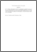# On the domination number of some graphs.

## Citation

Alikhani, Saeid and Peng, Yee Hock and Mohd Atan, Kamel Ariffin (2008) On the domination number of some graphs. International Mathematical Forum, 3 (38). pp. 1879-1884. ISSN 1312-7594

## Abstract

Let G = (V,E) be a simple graph. A set S ⊆ V is a dominating set of graph G, if every vertex in V − S is adjacent to at least one vertex in S. The domination number γ(G) is the minimum cardinality of a dominating set in G. It is well known that if e ∈ E(G), then γ(G−e)−1 ≤ γ(G) ≤ γ(G−e). In this paper, as an application of this inequality, we obtain the domination number of some certain graphs.Preview
PDF (Abstract)
on the domination number.pdfView Item